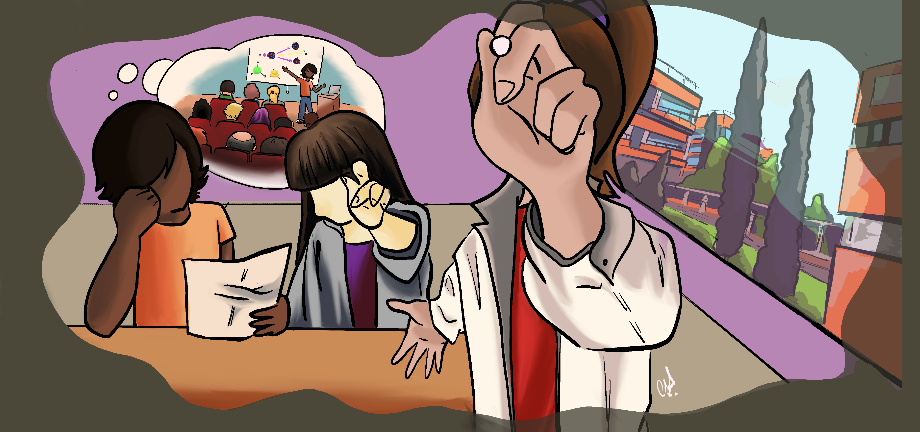#@FlipPhysics

21-25 March 2022
Salon de actos del IATA

## Thermal resonances and chiral symmetry restoration.

Not scheduled
15m
Salon de actos del IATA

#### Salon de actos del IATA

Carrer del Catedràtic Agustín Escardino Benlloch, 7, 46980 Paterna, Valencia
Talk

### Description

We analize the role played by the thermal $f_0(500)$ state or $\sigma$ in chiral symmetry restoration and propose an alternative sector (related with the thermal $K_0^*(700)$ or $\kappa$) to study $O(4)\times U_A(1)$ restoration. The temperature corrections to the spectral properties of those states are included in order to provide a better description of the scalar susceptibilities $\chi_S$ and $\chi_S^{\kappa}$ around the transition region. We use the Linear Sigma Model to establish the relation between $\chi_S$ and the $\sigma$ propagator, which is used as a benchmark to test the approach where $\chi_S$ is saturated by the $f_0(500)$ inverse self-energy. Within such saturation approach, a peak for $\chi_S$ around the chiral transition is obtained when considering the $f_0(500)$ generated as a $\pi\pi$ scattering pole within Unitarized Chiral Perturbation Theory at finite temperature. On the other hand, we show, using Ward Identities, that $\chi_S^{\kappa}$ develops a maximum above the QCD chiral transition, above which it degenerates with $\chi_{P}^{K}$ in the $O(4)\times U_A(1)$ restoration region. Such $\chi_S^{\kappa}$ peak can be described when it is saturated with the $K_0^*(700)$, which we compute in Unitarized Chiral Perturbation Theory through $\pi K$ scattering at finite temperature. That approach allows us in addition to examine the $\chi_S^{\kappa}$ dependence on the light- and strange-quark masses. Finally, a comparison with the Hadron Resonance Gas is also studied in this context.